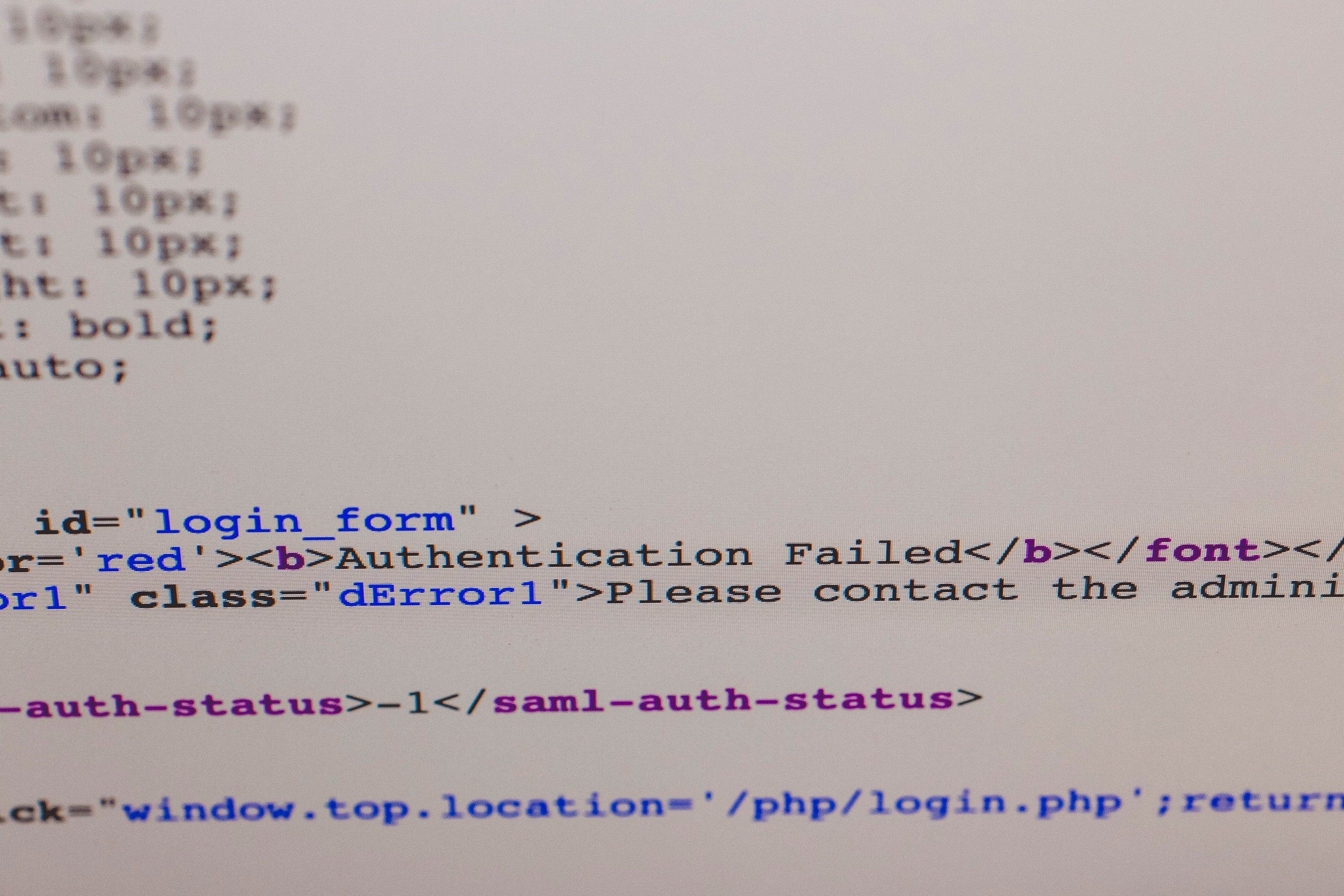Photo by Markus Spiske on Unsplash

# But, What Does A Digital Signature Actually Look Like?

I created the asecuritysite.com web site as I found there were very few sites that have practical implementations of the core methods. As a teacher, too, I believe that I should not give students something that I don’t know how to implement myself. And so the Web site has grown, as my knowledge of the field has grown. For me, it is a scratchpad for ideas, and where I want to show that the implementation of fairly complex cryptography methods is actually quite easy to understand. Along with this, things become real when they are made practical.

And so I get a good deal of questions, and one just said, “What does a digital signature actually look like?”.

Well, without going into detail, the most common digital signature is ECDSA (Elliptic Curve Digital Signature Algorithm). Basically it takes a message (M), a private key (sk) and a random value (k), and produces a digital signature of r and s. These are just two integer values. To check the signature, we take the message (M), the associated public key (pk), r and s, and perform a validation test, and, if it passes, the message has a correct signature.

So what does the signature actually look like? Is it just two numbers r and s that we add to the message? In many cases, it takes the form of the DER format, and which uses ASN.1 to define abstract types and values. Basically, it takes the two integer values (r and s) and encapsulates them into a more structured format. This format can be read by any computer system. One of the most basic types is SEQUENCE and is an ordered collection of one or more types. In DER, SEQUENCE is identified with a tag of “30”, and followed by a byte value for the length of the object defined. The other common types are OBJECT IDENTIFIER (and which has a tag of “06”), a BIT STRING (and which has a tag of “03”) and INTEGER (and which has a tag of “02”). In the case of a signature, we just use the INTEGER definition for the values.

So here is an example DER signature from NIST P-192 (and which uses 192-bit integer values) [here]:

`3035021900935f599bbdb30fc81a8b9de2f82311c6fa704838b53f9d7a0218267e3abb5bc3a44b0e368442ed3699b23ce87a28bc32cc53`

We first encounter the SEQUENCE (“30”), and then the next byte defines the length of the values which come next. In this case, “0x35” is 53 bytes. If you count the number of bytes after 35, you will find there are 53 bytes (or 106 hex characters) [here]:

`30 3502 19 00935f599bbdb30fc81a8b9de2f82311c6fa704838b53f9d7a02 18 267e3abb5bc3a44b0e368442ed3699b23ce87a28bc32cc53`

Next, we have a “02” tag, and then a “19”, and where the “19” value identifies 25 bytes (or 50 hex characters). We can then read r as the next 50 hex characters. Next, we have another “02” tag, and then a “19”, and where the “18” value identifies 24 bytes (or 48 hex characters). We can then read r as the next 48 hex characters. So, can we check the size of the integers produced? Well, we just multiply the number of bytes by 8, and we will determine this. Thus r is 25 bytes long, but the first byte is a zero, and s is 24 bytes, so the length of the values of r and s are 24 bytes long. This gives us 192 bits and which fits with the curve (P-192).

Now we will try a signature from a common curve (secp256k1) and which uses 256-bit values [here]:

`3046022100e4e87c417196c6e5cd63f93e94929ccda6d04fc0a7446922baf3070e854ec4f4022100a1ecd098008329de9bc93fb2ded6aaceecc921f7183d6b3cfc673b3ef8af219e`

Again we can parse, and notice that the integer values are longer this time [here]:

`30 46 02 21 00 e4e87c417196c6e5cd63f93e94929ccda6d04fc0a7446922baf3070e854ec4f402 21 00a1ecd098008329de9bc93fb2ded6aaceecc921f7183d6b3cfc673b3ef8af219e`

In fact, we have 0x21 bytes for the values of r and s, but the first byte is a zero, so we actually have 0x20 bytes, and which is 32 bytes. This will give us 256 bits, and which fits with the curve.

The code involves just parses for the DER format, and is [here]:

`import asn1import binasciifrom pem import class_id_to_string,tag_id_to_string,value_to_stringimport sysimport base64der='3046022100e4e87c417196c6e5cd63f93e94929ccda6d04fc0a7446922baf3070e854ec4f4022100a1ecd098008329de9bc93fb2ded6aaceecc921f7183d6b3cfc673b3ef8af219e'# See https://asecuritysite.com/encryption/sigs2indent=0if (len(sys.argv)>1):  der=str(sys.argv)def make_pem(st):  bff="-----BEGIN PUBLIC KEY-----\n"  bff=bff+base64.b64encode(st).decode()+"\n"  bff=bff+"-----END PUBLIC KEY-----\n"  print (bff)def read_pem(data):    """Read PEM formatted input."""    data = data.replace("\n","")    data = data.replace("","")    data = data.replace("-----BEGIN PUBLIC KEY-----","")    data = data.replace("-----END PUBLIC KEY-----","")    return binascii.hexlify(base64.b64decode(data))def show_asn1(string, indent=0):    while not string.eof():        tag = string.peek()        if tag.typ == asn1.Types.Primitive:            tag, value = string.read()            print(' ' * indent,end='')            print('[{}] {}: {}'.format(class_id_to_string(tag.cls), tag_id_to_string(tag.nr),value_to_string(tag.nr, value)))            if (tag.nr==4):               private_key=binascii.hexlify(value)              print(' ' * indent,end='')              print("Private key: ",private_key.decode())            if (tag.nr==3):                            res=binascii.hexlify(value).decode()              length=len(res)              if (res.__contains__('10001')):  # RSA                                rtn=res[1:].find("02")                print (res[rtn+3:rtn+5])                byte = int(res[rtn+3:rtn+5],16)-1                rtn=res[1:].find("00")                N=res[rtn+3:rtn+3+(byte)*2]                e=res[length-5:]                print(' ' * indent,end='')                print(f"RSA Modulus ({len(N)*4}) bits: {N}")                print(f"RSA e: {e}")              else :  # ECC                public_key_x=res[4:length//2]                public_key_y=res[length//2:]                print(' ' * indent,end='')                print(f"Public key ({public_key_x}, {public_key_y})")        elif tag.typ == asn1.Types.Constructed:            print(' ' * indent,end='')            print('[{}] {}'.format(class_id_to_string(tag.cls), tag_id_to_string(tag.nr)))            string.enter()            show_asn1(string, indent + 2)            string.leave()Print=Trueif (len(der)>500): Print=Falseif (der.__contains__("BEGIN")):  print("Found PEM")  der=read_pem(der)if (Print): print (f"PEM: {der}\n")st=binascii.unhexlify(der)decoder = asn1.Decoder()decoder.start(st)show_asn1(decoder)print()if (Print): make_pem(st)`

And there you go … the magic of (r,s).

Professor of Cryptography. Serial innovator. Believer in fairness, justice & freedom. EU Citizen. Auld Reekie native. Old World Breaker. New World Creator.

Professor of Cryptography. Serial innovator. Believer in fairness, justice & freedom. EU Citizen. Auld Reekie native. Old World Breaker. New World Creator.